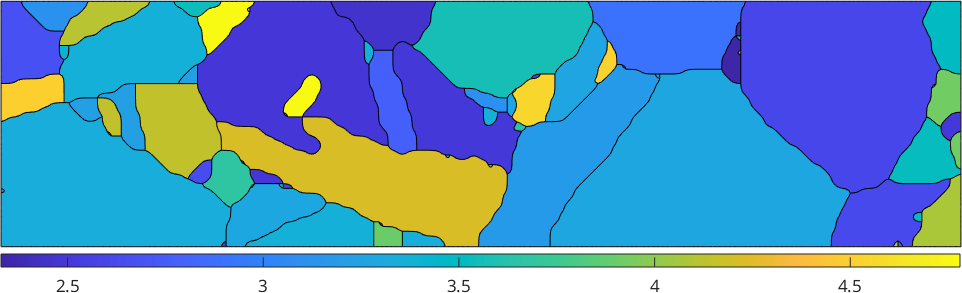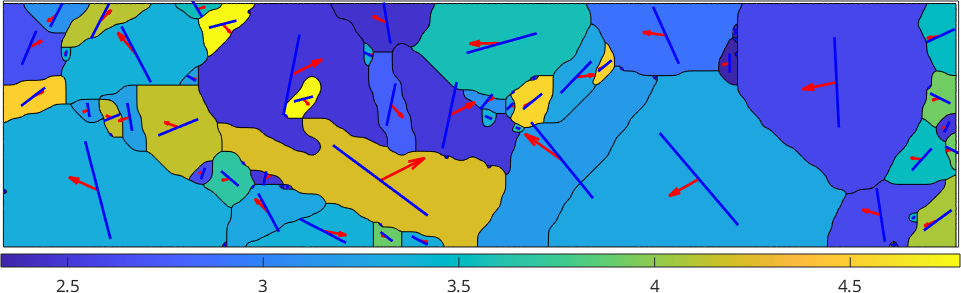script_PlasticDeformation edit page

## Schmid Factor Analysis

This script describes how to analyze Schmid factors.

Let us assume a Nickel crystal

Since Nickel is fcc a dominant slip system is given by the slip plane normal

and the slip direction (which needs to be orthogonal)

For tension in direction 123

the Schmid factor for the slip system [0-11](111) is defined by

The same computation can be performed by defining the slip system as an MTEX variable

and using the command SchmidFactor

Ommiting the tension direction r the command SchmidFactor returns the Schmid factor as a spherical function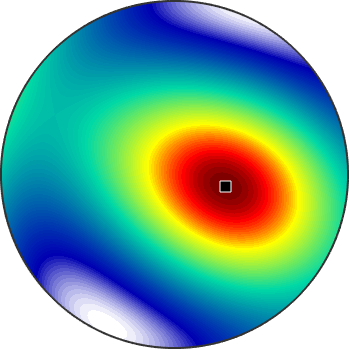## Stress Tensor

Instead by the tension direction the stress might be specified by a stress tensor

Then the Schmid factor for the slip system sS and the stress tensor sigma is computed by

## Active Slip System

In general a crystal contains not only one slip system but at least all symmetrically equivalent ones. Those can be computed with

The option antipodal indicates that Burgers vectors in oposite direction should not be distinguished. Now

returns a list of Schmid factors and we can find the slip system with the largest Schmid factor using

The above computation can be easily extended to a list of tension directions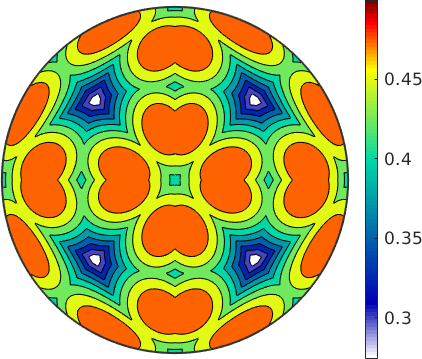We may also plot the index of the active slip system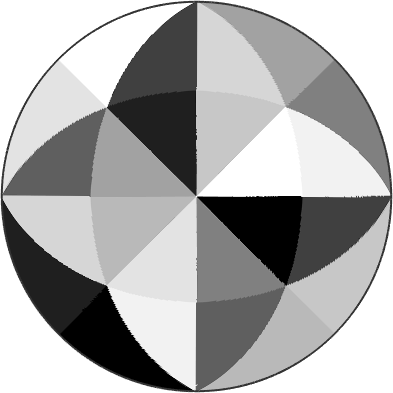and observe that within the fundamental sectors the active slip system remains the same. We can even visualize the the plane normal and the slip direction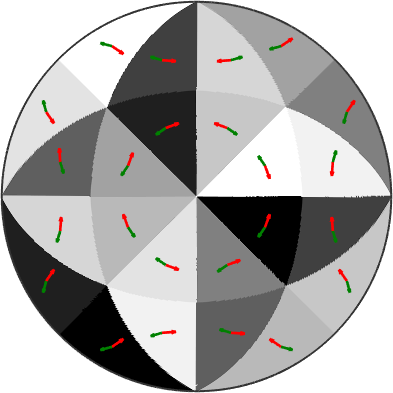If we perform this computation in terms of spherical functions we obtain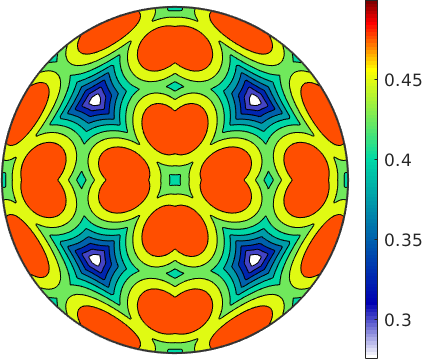## The Schmid factor for EBSD data

So far we have always assumed that the stress tensor is already given relatively to the crystal coordinate system. Next, we want to examine the case where the stress is given in specimen coordinates and we know the orientation of the crystal. Lets import some EBSD data and computet the grains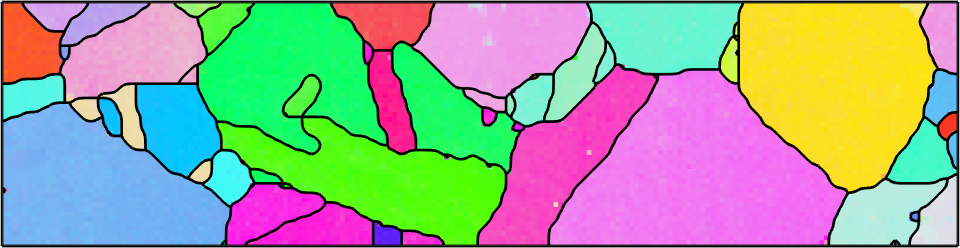We want to consider the following slip systems

Since, those slip systems are in crystal coordinates but the stress tensor is in specimen coordinates we either have to rotate the slip systems into specimen coordinates or the stress tensor into crystal coordinates. In the following sections we will demonstrate both ways. Lets start with the first one

These slip systems are now arranged in matrix form where the rows corrspond to the crystal reference frames of the different grains and the rows are the symmetrically equivalent slip systems. Computing the Schmid faktor we end up with a matrix of the same size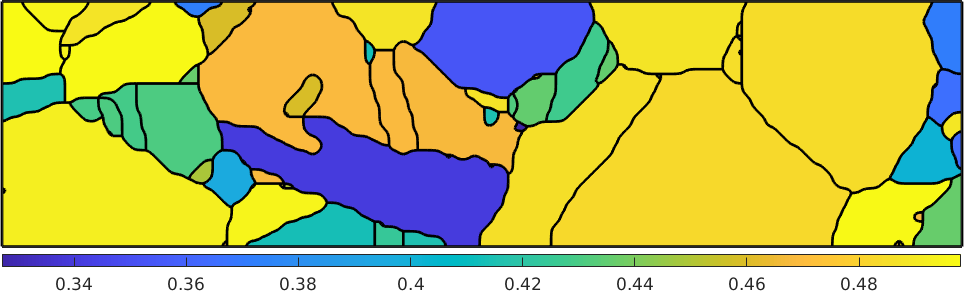Next we want to visualize the active slip systems.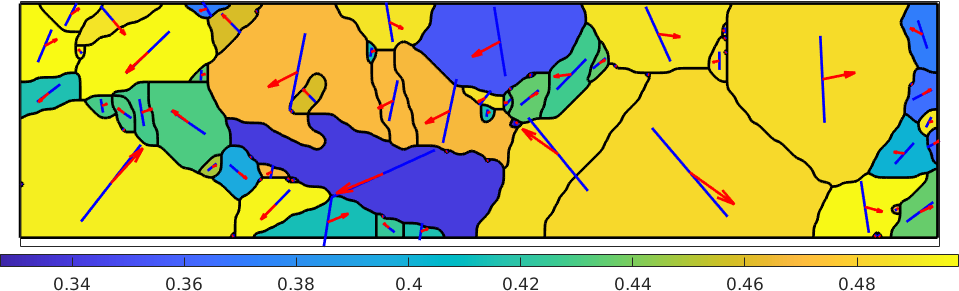We observe that the Burgers vector is in most case aligned with the trace. In those cases where trace and Burgers vector are not aligned the slip plane is not perpendicular to the surface and the Burgers vector sticks out of the surface.

Next we want to demonstrate the alternative route

This becomes a list of stress tensors with respect to crystal coordinates - one for each grain. Now we have both the slip systems as well as the stress tensor in crystal coordiantes and can compute the Schmid factor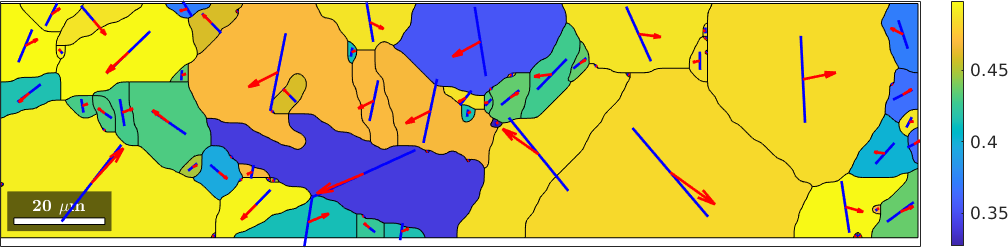## Strain based analysis on the same data set